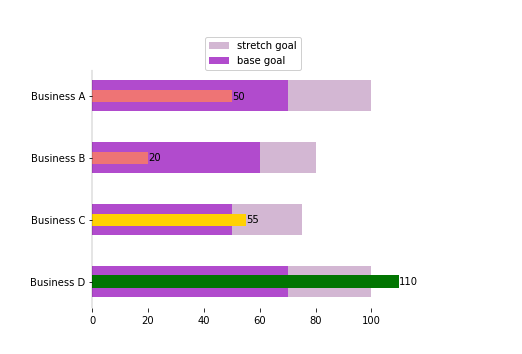# Python Bullet ChartsI've enjoyed creating bullet charts in Periscope using the technique outlined in this community post here. However, if we seek to further customize the look of a bullet chart, Periscope's R/Python integration comes in very handy! The above image is a bullet chart created using matplotlib, and allows quick interpretation of how well a business is tracking toward its goals.

Inputs:

• df : a dataframe with 4 columns
• base goal - a numerical value of the quota/base goal
• stretch goal - a numerical value of the target/stretch goal
• current value
• color_code: an optional boolean parameter that color codes the current value based on whether the base and stretch goals have been met. Default value set to False

Snippet:

```# SQL output is imported as a pandas dataframe variable called "df"
import pandas as pd
import matplotlib.pyplot as plt
import numpy as np

# Function: bullet_chart, creates a horizontal bar graph representing a bullet chart
# Inputs: (1) dataframe with 4 rows - the name of the column ("business"), "base goal" or the quota, "stretch goal" or the target, and the "current value" (2) Optional boolean paramater that shades the current value green/yellow/red based on whether the base/stretch goals have been met
# Output: matplotlib image representing a bullet chart
def bullet_chart(df,color_code=False):
y_pos = np.arange(len(df.index))

#assign coloring
df["col"]="indigo"
if (color_code==True):
for i in y_pos:
if(df["current value"][i]>=df["stretch goal"][i]):
df["col"][i]="green"
elif(df["current value"][i]>=df["base goal"][i]):
df["col"][i]="gold"
else:
df["col"][i]="lightcoral"

#Initialize plot
fig, ax = plt.subplots()
ax.barh(y_pos, df["stretch goal"], height=0.5, align='center', color='thistle', label="stretch goal")
ax.barh(y_pos, df["base goal"], height=0.5, align='center', color='mediumorchid', label = "base goal")
ax.barh(y_pos, df["current value"], height=0.2, align='center',color=df["col"])
ax.set_yticks(y_pos)
ax.invert_yaxis()

for i in y_pos:
ax.text(df["current value"][i], i+0.05, df["current value"][i])

plt.legend(loc=(0.35,1.0))
ax.spines['left'].set_linewidth(0.2)
ax.spines['bottom'].set_linewidth(0)
ax.spines['right'].set_linewidth(0)
ax.spines['top'].set_linewidth(0)